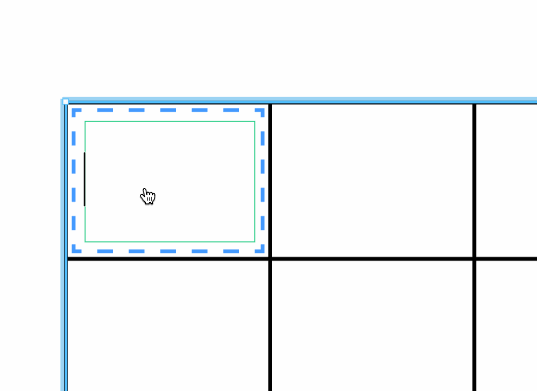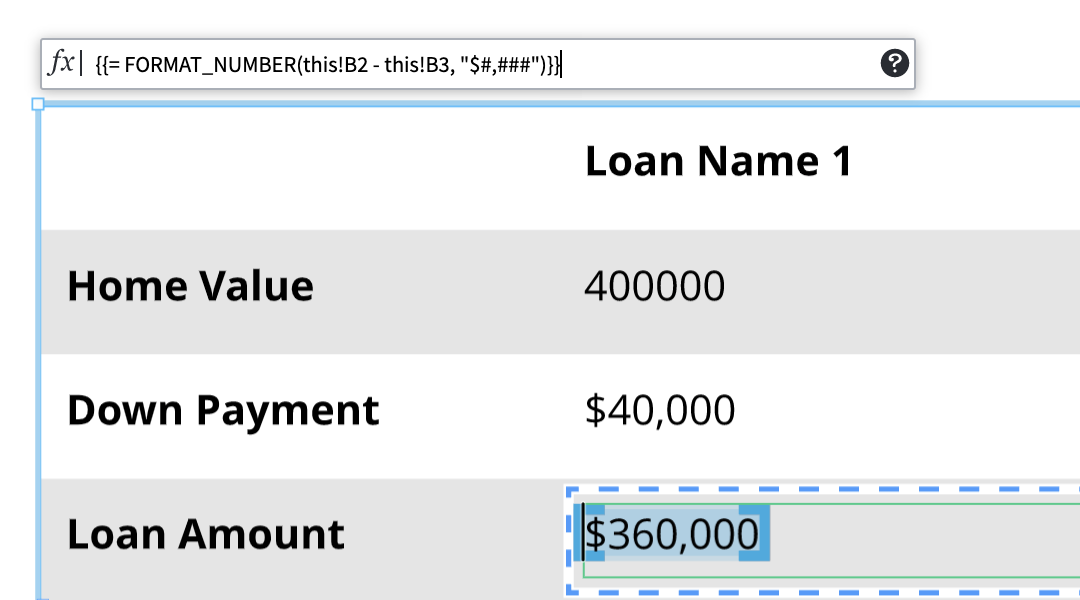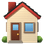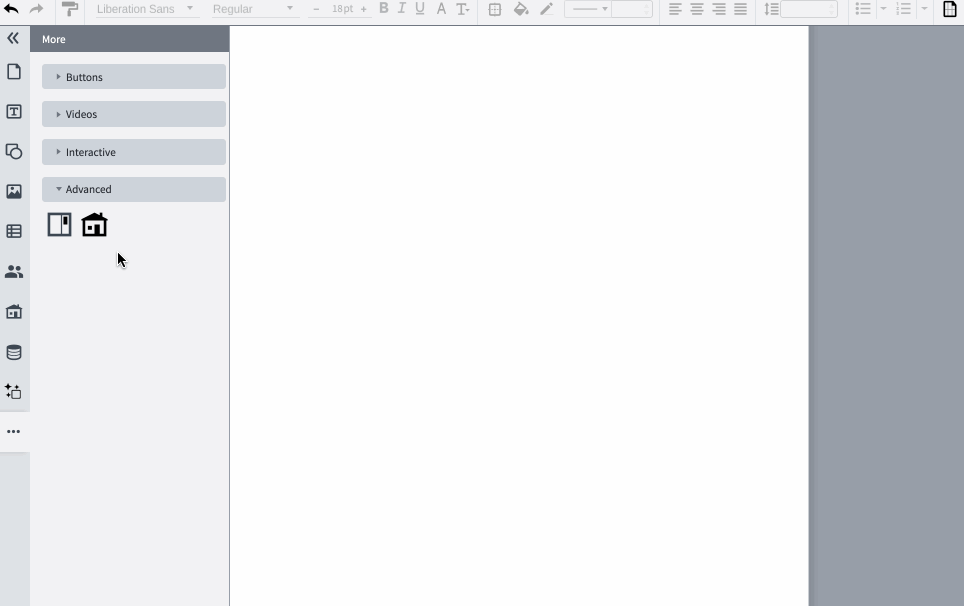# How to use formulas

## Similar to Excel and Google Sheets, formulas in Marq allow you to automate calculations in a text box, shape, or table cell.

Create a formula in a cell, text box, or shape:

Formulas are created by typing "{ {=" and then closing it off with a "} }"

Ex: Type

Once you close off the formula, you can edit the formula in the formula bar that will appear at the top of the object#### Create a formula referencing other cells:

Use "this!" in your formula and reference a cell.
Ex: Type

Note: you can only reference cells within the object/table you have selected.#### Format a formula

Type the format you want to use, use the formula, { {= FORMAT_NUMBER{[insert formula, "[insert format")} }

Ex to format a comma and a \$:Marq rounds out its table data capabilities by offering formulas to users. Much the way spreadsheet tools allow users to analyze, adjust, and quantify their data, Marq allows users to run certain formulas on their projects.

Go to the left side bar -> ... -> Advanced ->that creates a mortgage calculator shape, which has cells with formulas.#### Glossary

• Collection: A single sheet (tab) in an imported spreadsheet data set.
• Reference Key: The column in a collection that uniquely identifies a row. For example, each person has a unique SSN but may not have a unique name. The SSN 123-01-4567 uniquely refers to the person "John Albert Smith", but the name "John Smith" could refer to thousands of people.
• Custom Data: Shape data that is added manually, rather than referenced by a Reference Key.
• Label and Value: Shape data comes in Label: Value pairs. For example, "Name: John Smith" has the label "Name" and value "John Smith"
• Element: Shape, line, page, group, etc. in Marq
• Keyed Values: Shape data that is referenced by a Reference Key
• Parent: The Marq group to which an element belongs. Does not refer to elements upstream or downstream.
• Child: An element of the selected group or page. Does not refer to elements upstream or downstream.
• Descendant: The children and all of their children and all of their children, etc.

#### Example Formulas

Marq Formulas: Data References
• Shorthand to reference 'this current shape' when getting data properties.
• =@'Property 1' returns whatever the value of the property called 'Property 1' on the current shape is.
• ex: =@'Total' → returns 10, when the current shape has a property called 'Total' that equals 10.
LABEL
• Gets the unique shape id for the shape it references
• =LABEL(this), =LABEL(parent)
• ex: =LABEL(this) → ab3756dhty!975 (returns the shape ID, unique to each shape)
• Why? You can use this to then listen to specific shapes!
• =#'shape_id'."Property Name" → will give you the value of "Property Name" from the shape whose ID you have passed!
• ex: =#'shape_id'."Total" → 250. Then you can do something to this shape, based on the other shape's Total Value
[] or INDEX
• Allows you to access the value at a given position in a list (must pass in the numbered position, starting at 1 - does not function as a 0-indexed list)
• Works with deeply nested arrays!!
• =list[index position] → returns the value at the given index of the list
• =INDEX(list, index position) → returns the value at the given index of the given list
• ex: =CHILDREN.'Employee Name' → returns the 3rd employee name in the list
• ex: =INDEX(CHILDREN.'Employee Name', 3) → returns the 3rd employee name in the list
• ex: =INDEX(INDEX(DOWNSTREAMDEEP.'Connected', 2), 3) → returns the 2nd item from the list of connected items, of the 2nd item that is deeply downstream of the current shape.
• At index 1 contains a list of everything connected to shape B, which are shapes [A, C]
• At index 2 contains a list of everything connected to shape C, which are shapes [B, 1, 2, 3]
• At index 3-5, contains a list of everything connected to shapes 1, 2, and 3 respectively, which in each case is just shape C.
• So now, INDEX gets us the second item (index 2) of things deeply downstream of shape A - which is the list of "everything connected to shape C" → [B, 1, 2, 3]
• Then, the outer INDEX gets us the third item (index 3) of the list of shapes connected to shape C [B, 1, 2, 3] → 2
• So, we get 2.
• In this case, you give the position of the list that you want within the current list, and then give the position of the item in the nested list.
• Note that this nesting will work inside out, evaluating the most deeply nested terms first. So first, it gets us the CONNECTED shapes of everything deeply downstream of shape A.
• A is upstream of B, B is upstream of C, and C is upstream of 1, 2, and 3. On shape A, DOWNSTREAMDEEP.CONNECTED = [ [A, C], [B, 1, 2, 3], C, C, C ]
• This also works with the [] : =DOWNSTREAMDEEP.CONNECTED → returns 2
FIELDLOOKUP
• Uses a reference key and the collection name to find a data property.
• =FIELDLOOKUP("Collection Name", "Shape Data Label", "Ref Key")
• ex: =FIELDLOOKUP("Sheet1", "Employee Name", "123") → gives us the name of the employee who on Sheet1, has the ref key 123
LOOKUP
• Finds data from a collection, by reference key, and returns an array of all the data associated with that reference key.
• If you add a shape data property name (ex: column header), then it will give you that specific property.
• =LOOKUP("Collection Name", "Reference Key") → will return ["All", "data", "from", "the", "row", "with", "the", "reference", "key"] as a list
• =LOOKUP("Collection Name", "Reference Key")."Property Name" → will return "Value associated with that specific property"
• ex: =LOOKUP("Sheet1", "123") → ["123", "Steve Rogers", "Captain America", "starspangledman@gmail.com"]
• ex: =LOOKUP("Sheet1", "123")."Name" → "Steve Rogers"
Marq Formulas: String Manipulation
• Concatenates strings.
• ='First String' & 'Second String' → returns 'First StringSecond String'
• ex: ='Hello' & 'There' → returns 'HelloThere'
• ex: ='Hello ' & 'There' → returns 'Hello There'
• ex: ='Hello' & ' ' & 'There' → returns 'Hello There'
TRIM
• Trims trailing whitespace from the string passed in (text passed in) =TRIM(string)
• =TRIM(" hi ") → "hi"
• =TRIM("I love chocolate! ") → "I love chocolate!"
Marq Formulas: Number Manipulation
+, -, *, /
• Typical Math operators behave as expected for addition, subtraction, multiplication, and division.
• =1+2 → 3 etc.
• Note: modulus (%) as seen in JS does not work!
PRODUCT
• Gives the product of either an array or all numbers passed in with commas.
• If a complex array, like children. children flattens the array to calculate.
• =PRODUCT(children."Property 1")
• =PRODUCT(1, 2, 3, 4, 5, 6, 7) → 1 * 2 * 3 * 4 * 5 * 6 * 7 → 5040
ASPERCENT
• Takes a number and turns it into a Percent.
• =ASPERCENT(num) → returns num as a %
• ex: =ASPERCENT(.1) → 10%
• ex: =ASPERCENT(.158) → 15.8%
• ex: =ASPERCENT(1.4) → 140%
ROUND
• Rounds a number to a specified number of digits - default is 0.
• =ROUND(number, number of digits to round to)
• ex: =ROUND(5.6978695869) → 6
• ex: =ROUND(5.6978695869, 1) → 5.7
• ex: =ROUND(5.6978695869, 2) → 5.70
<, <=, =, >=, >, <>
• Typical math & comparison operators work as expected for less than, less than or equal to, equal to, greater than or equal to, greater than, and not equal to.
• ex: =5 < 10 → returns true
• ex: =6 = 7 → returns false
• ex: ='Hi' = 'Hi' → returns true (note: follows strict equivalency, so 'Hi' = 'hi' will be false!)
• ex: =@'Property 1' > page.'Property 1' → will return true or false
• ex: =5 <> 10 → returns true (5 is not equal to 10)
AND
• Resolves to true if all of the given expressions/values are true, and false otherwise
• =AND("expression", "expression2",....)
• ex: =AND(this."Property 1" = 10, this."Property 2" > 5)
OR
• Resolves to true if any of the given expressions/values are true, and false if none are true
• =OR("expression", "expression2", "expression3", ....)
• ex: =OR(this."Property 1" = 10, this."Property 2" > 5)
NOT
• Resolves to true if the given expression/values is false, and false if the given expression is true
• =NOT("expression")
• ex: =NOT(10 < 1) → 10 < 1 is a false statement, so the function will read this as =NOT(false), which resolves to true
• ex: =NOT(10 > 1) → 10 > 1 is a true statement, so the function will read this as =NOT(true), which resolves to false
IF
• Resolves to one value, if the statement give, is true, and to the second value if it is false
• =IF("expression", "value if true", "value if false")
• ex: =IF(10 < 20, "Yes", "No") → resolves to "Yes" because 10 is less than 20.
• ex: =IF(10 > 20, "Yes", "No") → resolves to "No" because 10 is not greater than 20.
• this.A = 10
• this.B = 15
• this.C = 12
• Nested If ex:
• =IF(this.A > this.B, this.A, IF(this.B > this.C, this.B, this.C)) →
• =IF(15 > 12, 15, 12) → 15 is greater than 12, so the answer resolves to 15
• =IF(10 > 15, 10, IF(15 > 12, 15, 12)) → the first if, 10 > 15, is false, so now we focus on the second if statement
ISEMPTY
• Returns true if the property value is empty
• =ISEMPTY(this."Property 1")
ISNOTEMPTY
• Returns true if the property value is not empty
• =ISNOTEMPTY(this."Property 1")
String/Number Checks
ISODD
• Checks if a number is odd. Resolves to true if odd, false if even.
• =ISODD("number or value")
• =ISODD(9) → true
• =ISODD(10) → false
ISEVEN
• Checks if a number is even. Resolves to true if even, false if odd.
• =ISEVEN("number or value")
• =ISEVEN(9) → false
• =ISEVEN(10) → true
• CONTAINS
• Checks to see if a property or string contains a value (true if yes, false if no)
• =CONTAINS("Value you are checking", "what you want to know if it contains")
• ex: =CONTAINS(this."Property 1", "abc") → resolves to true if Property 1 contains "abc", and false otherwise
DOESNOTCONTAIN
• Checks to see if a property or string does NOT contain a value (true if it lacks the value, false if it does contain the value)
• =DOESNOTCONTAIN("Value you are checking", "what you want to know if it contains")
• ex: =DOESNOTCONTAIN(this."Property 1", "abc") → resolves to false if Property 1 contains "abc", resolves to true if Property 1 does not have "abc" in it
STARTSWITH
• Checks if a property or string starts with a certain value
• ex: =STARTSWITH(this."Property 1", "s") → resolves to true if Property 1 starts with "s"
ENDSWITH
• Checks if a property or string ends with a certain value
• =ENDSWITH("Value you are checking", "what it might end with")
• ex: =ENDSWITH(this."Property 1", "s") → resolves to true if Property 1 ends with "s"
BETWEEN
• Checks if a number is between two other numbers (inclusive) → true if it IS between those numbers, and false if it is NOT between those numbers.
• =BETWEEN("number we are checking", "lower bound inclusive", "upper bound inclusive")
• ex: =BETWEEN(14, 12, 15) → resolves to true because 14 is between 12 and 15, inclusive
• ex: =BETWEEN(11, 12, 15) → resolves to false because 11 is not between 12 and 15, inclusive
• ex: =BETWEEN(12, 12, 15) → resolves to true because 12 is between 12 and 15, inclusive
NOTBETWEEN
• Checks if a number is not between two other numbers (inclusive) → true if it is NOT between those numbers, and false if it IS between those numbers.
• =NOTBETWEEN("number we are checking", "lower bound inclusive", "upper bound inclusive")
• ex: =NOTBETWEEN(14, 12, 15) → resolves to false because 14 is between 12 and 15, inclusive
• ex: =NOTBETWEEN(11, 12, 15) → resolves to true because 11 is not between 12 and 15, inclusive
• ex: =NOTBETWEEN(12, 12, 15) → resolves to false because 12 is between 12 and 15, inclusive
Marq Formulas: Analytical Functions
SUM
• Gives the sum of either an array or all numbers passed in with commas.
• =SUM(children."Property 1")
• =SUM(1, 2, 3, 4, 5, 6, 7)
MEAN
• Gives the mean of either an array or all numbers passed in with commas.
• =MEAN(children."Property 1")
• =MEAN(1, 2, 3, 4, 5, 6, 7)
MAX
• Gives the max of either an array or all numbers passed in with commas.
• =MAX(children."Property 1")
• =MAX(1, 2, 3, 4, 5, 6, 7)
MIN
• Gives the min of either an array or all numbers passed in with commas.
• =MIN(children."Property 1")
• =MIN(1, 2, 3, 4, 5, 6, 7)
COUNT
• Count the number of elements you pass in (a list or a reference to a list) =COUNT(list)
• ex: =COUNT([a, b, c, d, e, f]) → 6
• ex: =COUNT(children) → returns however many children the current shape/space has
COUNTIF
• Count the number of elements passed in that meet the specified condition
• =COUNTIF([1,2,3,4,5], THIS < 3) → returns 2 (only 1 and 2 are less than 3)
• =COUNTIF(children, THIS."Total" < 10) → returns however many of the children have a value "Total" that is less than 10
• =COUNTIF(children, contains(THIS."Name", "Steve") → returns however many of the children have "Steve" in their name.
FILTER
• Given a list, this filters the list down to just contain the items that match the condition in the second parameter.
• =FILTER(list, condition to keep in the list)
• ex: =FILTER(descendants, contains(@'Employee Name', 'Steve')) → returns a list of every descendant of the space that has the property 'Employee Name' that also contains 'Steve'.
• ex: =FILTER(@'Accounts', @'Value' < 5000) → given that the property 'Accounts' contains all the accounts, returns on the accounts that have a value of less than 5000.
SORT
• Given a list, this sorts the list in ascending order.
• Optional parameters allow you to change the sort.
• =SORT(list)
• ex: =SORT(children) → returns a list of the children of the space, such as the page, sorted by their text, such as [Amsterdam, London, Rome].
• ex: =SORT(downstream) → given that all the downstream shapes contain a name, returns those shape references sorted by their text (the names), such as [Amanda, Joe, Zoe].
• Adding in another optional parameter, that comes after 'asc'/'desc', for sorting shapes by a specific property:
• =SORT(list, order, sortBy) → sorts the list in the given order, by the given property.
• ex: =SORT(children, 'asc', this.'City') → given that the children of the space have a property called 'City', returns a list of the children of the space, sorted by their 'City' property in ascending order, such as [Amsterdam, London, Rome].
• ex: =SORT(children, 'desc', this.'Shipping Date') → given that the children of the space have a property called 'Shipping Date', returns a list of the children of the space, sorted by their 'Shipping Date' property in descending order, such as [London, Amsterdam, Rome] → indicating that London's shipping date is the latest, and Rome's shipping date is the soonest.
• ex: =SORT(children, 'desc', this.'Current ARR') → given that the children of the space have a property called 'Current ARR', returns a list of the children of the space, sorted by their 'Current ARR' property in descending order, such as [Account #3, Account #8, Account#2] → indicating that the largest account is Account #3, followed by Account #8, etc.
• Adding in the optional parameter, for sorting by ascending (A to Z, smallest value first), or by descending (Z to A, largest value first).
• =SORT(list, 'asc') → sorts the list in an ascending order
• =SORT(list, 'desc') → sorts the list in an descending order
• ex: =SORT(children, 'asc') → returns a list of the children of the space, such as the page, sorted by their text in ascending order, such as [Amsterdam, London, Rome].
• ex: =SORT(children, 'desc') → returns a list of the children of the space, such as the page, sorted by their text in ascending order, such as [Rome, London, Amsterdam].
• ex: =SORT(downstream, 'asc') → given that all the downstream shapes contain a name, returns those shape references sorted by their text (the names) in ascending order, such as [Amanda, Joe, Zoe].
• ex: =SORT(downstream, 'desc') → given that all the downstream shapes contain a name, returns those shape references sorted by their text (the names) in descending order, such as [Zoe, Joe, Amanda].
• ex: =SORT(downstreamdeep.'Performance Review', 'desc') → given that all the downstream shapes contain a property called 'Performance Review', returns a list of the deeply downstream shapes' performance review scores, sorted by in descending order, such as [7, 6, 6, 5, 4, 4, 4, 3, 2].
Marq Formulas: Smart Containers
CONTENTS
• Reference (or retrieve) the contents of a Smart Container
• =COUNT(CONTENTS) → returns 3 (if there are three items in the container)
• =SUM(CONTENTS.Planned) → Returns Sum of all items Planned values inside a container
CONTAINER
• Reference the container that contains an item in Smart Containers.
• Used on an item in a smart container.
• =CONTAINER → returns an item's container label
• =IF(CONTAINER = 'Low') → Returns all items whose container is labeled 'Low'
FILLCOLOR / BACKGROUNDCOLOR
• Takes in a referenced object (this, parent, upstream, downstream, page, etc.) and returns the hex fill color.
• =BACKGROUNDCOLOR(thing I want the color of)
• =FILLCOLOR() returns the shape's fill color
• ex: =BACKGROUNDCOLOR(page) → #FFFFFF
CURRENTSECOND
• A counter that starts at the current second in real time and counts up every second. =CURRENTSECOND
• Resets at 60
IFERROR
• If there is an error in the expression provided, this returns the value that you want if there is an error.
• The purpose is to keep your project from breaking if you get an error!
• =IFERROR(expression, value if the expression produces an error)
• =IFERROR(parent."Total", 0) → returns 0 if, for example, this is on a shape that does not have a parent or if the parent does not have a property called "Total"